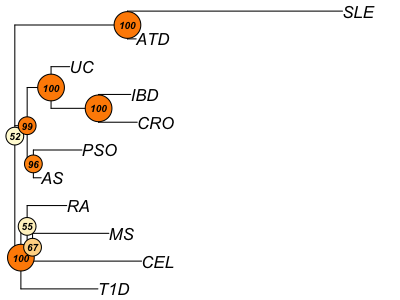# 2 Summary

This demo showcases the analytic power of using XGR to make sense of GWAS SNPs, including network analysis, enrichment analysis, and annotation analysis. Network analysis supported in XGR is unique in its power to identify SNP-modulated gene networks (via SNP scoring, gene scoring and network scoring) and to perform cross-disease analysis. When coupled with annotation analysis (via looking at nearby gene annotations by ontologies or via looking at co-localised functional genomic annotations), XGR is able to perform in-depth interpretations of the underlying genetic landscape of immunological diseases based on GWAS summary data.

# 3 Packages

``````library(XGR)

# Specify the locations of built-in data

# 4 Data

The dataset `ImmunoBase` is GWAS lead SNPs associated with immunologically related human diseases, obtained from `ImmunoBase`.

``````ImmunoBase <- xRDataLoader(RData.customised='ImmunoBase', RData.location=RData.location)
disease_list <- lapply(ImmunoBase, function(x) x\$disease)
# get the number of disease associated variants/SNPs
variants_list <- lapply(ImmunoBase, function(x) length(unique(names(x\$variants))))
# get the number of genes that are located within 50kb distance window of SNPs
genes_list <- lapply(ImmunoBase, function(x) length(x\$genes_variants<=50000))
# create a data frame
df_ib <- data.frame(Code=names(ImmunoBase), Disease=unlist(disease_list), num_SNPs=unlist(variants_list), num_nearby_genes=unlist(genes_list), stringsAsFactors=F)``````

We restrict common diseases and each having at least 30 GWAS lead SNPs. As such we have 11 common diseases for cross-disease analysis below.

``````ind <- match(c('IBD','CRO','UC','PSO','AS','RA','CEL','T1D','MS','SLE','ATD'), names(ImmunoBase))
IB <- ImmunoBase[ind]``````

A summary of 11 diseases (along with GWAS lead SNPs and their nearby genes):

Code Disease num_SNPs num_nearby_genes
IBD Inflammatory Bowel Disease (IBD) 148 2280
CRO Crohn’s Disease (CRO) 174 1945
UC Ulcerative Colitis (UC) 120 1651
PSO Psoriasis (PSO) 68 624
AS Ankylosing Spondylitis (AS) 32 437
RA Rheumatoid Arthritis (RA) 165 1070
CEL Celiac Disease (CEL) 144 734
T1D Type 1 Diabetes (T1D) 180 1046
MS Multiple Sclerosis (MS) 237 1725
SLE Systemic Lupus Erythematosus (SLE) 61 742
ATD Autoimmune Thyroid Disease (ATD) 33 233

# 5 Showcase

## 5.1 Scoring from SNPs to genes

Do SNP scoring and gene scoring for each disease

``````disease_ls <- lapply(IB, function(x){
gr <- x\$variant
data <- GenomicRanges::mcols(gr)[, c('Variant','Pvalue')]
xSNP2GeneScores(data=data, include.LD='EUR', LD.r2=0.8, significance.threshold=5e-8, distance.max=50000, decay.kernel="rapid", decay.exponent=2, GR.SNP="dbSNP_GWAS", GR.Gene="UCSC_knownCanonical", scoring.scheme="max", verbose=T, RData.location=RData.location)
})``````

Get all diseases and all genes

``````## all diseases
allDiseases <- names(IB)
## all Genes
res_Gene <- lapply(disease_ls, function(x) x\$Gene\$Gene)
allGenes <- unique(unlist(res_Gene))``````

A matrix of Genes X Diseases:

``````mat_Gene <- 0
for(i in 1:length(disease_ls)){
df <- disease_ls[[i]]\$Gene
df\$Disease <- rep(allDiseases[i], nrow(df))
mat <- xSparseMatrix(df[,c("Gene","Disease","Score")], rows=allGenes, columns=allDiseases)
mat_Gene <- mat_Gene + mat
}
mat_Gene <- as.matrix(mat_Gene)``````

A neighbor-joining tree (with bootstrap confidence values) based on the matrix of Genes X Diseases. The distance between two diseases is defined as the sum of their difference in gene scores (that is, manhattan/cityblock distance).

``````data <- mat_Gene
tree_bs <- visTreeBootstrap(data=t(data), algorithm="nj", metric="manhattan", num.bootstrap=10000, reroot="min.bootstrap", visTree=T, nodelabels.arg=list(cex=0.6))``````The corresponding consensus tree of the above bootstrapped tree is (based on the majority voting):

``````data <- mat_Gene
tree_cons <- visTreeBootstrap(data=t(data), algorithm="nj", metric="manhattan", num.bootstrap=10000, consensus=T, reroot="min.bootstrap", visTree=T)
g <- igraph::as.igraph(tree_cons)
vertex.label <- V(g)\$name
vertex.label <- gsub("Node.*", "", vertex.label)
vertex.size <- rep(12, vcount(g))
vertex.size[vertex.label==''] <- 2
xVisNet(g=g, glayout=layout_with_kk, vertex.shape="sphere", vertex.size=vertex.size, vertex.color="white", vertex.label=vertex.label, vertex.label.dist=0.9, vertex.label.font=2, , newpage=F)``````